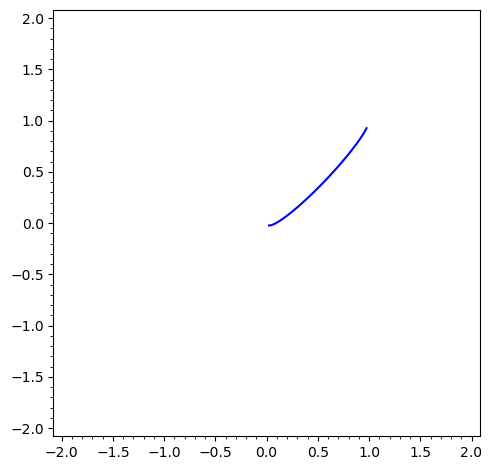# Solve an equation with variable under square-root

I want to solve $p=h+\sqrt{\dfrac{p\cdot\left(1-p\right)}{n}}$ for $p$.

This works with
In:

eqn = (p-h)^2==p*(1-p)/n
solve(eqn, p)


Out:

[p == 1/2*(2*h*n - sqrt(-4*(h^2 - h)*n + 1) + 1)/(n + 1), p == 1/2*(2*h*n + sqrt(-4*(h^2 - h)*n + 1) + 1)/(n + 1)]


But this works not with

In:

eqn1 = p-h==sqrt(p*(1-p)/n)
solve(eqn1, p)


Out:

[p == h + sqrt(-(p^2 - p)/n)]


How can I solve the root-equation directly?

edit retag close merge delete

Sort by » oldest newest most voted

NOTE : Incomplete answer : solution framework, suitable for numerical computation (any precision), but no formal solution.

If two quantities are equal, their squares are also equal. You can therefore get candidate solutions to eqn by solving eqn.lhs()^2==eqn.rhs()^2, which van be abbreviated as eqn^2.

But by doing this, you may introduce spurious solutions : x==y implies that x^2==y^2, but so does x==-y. So you must filter your set of candidate solutions by checking against eqn.

sage: (eqn^2).solve(p)
[p == 1/2*(2*h*n - sqrt(-4*(h^2 - h)*n + 1) + 1)/(n + 1), p == 1/2*(2*h*n + sqrt(-4*(h^2 - h)*n + 1) + 1)/(n + 1)]


$$\left[p = \frac{2 \, h n - \sqrt{-4 \, {\left(h^{2} - h\right)} n + 1} + 1}{2 \, {\left(n + 1\right)}}, \quad p = \frac{2 \, h n + \sqrt{-4 \, {\left(h^{2} - h\right)} n + 1} + 1}{2 \, {\left(n + 1\right)}}\right]$$

but formally checking these two roots isn't specially easy (numerically, it's a piece of cake...) : it amounts to check that :

sage: [eqn.subs(u) for u in (eqn^2).solve(p)]
[-h + 1/2*(2*h*n - sqrt(-4*(h^2 - h)*n + 1) + 1)/(n + 1) == 1/2*sqrt(-(2*h*n - sqrt(-4*(h^2 - h)*n + 1) + 1)*((2*h*n - sqrt(-4*(h^2 - h)*n + 1) + 1)/(n + 1) - 2)/((n + 1)*n)),
-h + 1/2*(2*h*n + sqrt(-4*(h^2 - h)*n + 1) + 1)/(n + 1) == 1/2*sqrt(-(2*h*n + sqrt(-4*(h^2 - h)*n + 1) + 1)*((2*h*n + sqrt(-4*(h^2 - h)*n + 1) + 1)/(n + 1) - 2)/((n + 1)*n))]


$$-h + \frac{2 \, h n - \sqrt{-4 \, {\left(h^{2} - h\right)} n + 1} + 1}{2 \, {\left(n + 1\right)}} = \frac{1}{2} \, \sqrt{-\frac{{\left(2 \, h n - \sqrt{-4 \, {\left(h^{2} - h\right)} n + 1} + 1\right)} {\left(\frac{2 \, h n - \sqrt{-4 \, {\left(h^{2} - h\right)} n + 1} + 1}{n + 1} - 2\right)}}{{\left(n + 1\right)} n}}$$

$$-h + \frac{2 \, h n + \sqrt{-4 \, {\left(h^{2} - h\right)} n + 1} + 1}{2 \, {\left(n + 1\right)}} = \frac{1}{2} \, \sqrt{-\frac{{\left(2 \, h n + \sqrt{-4 \, {\left(h^{2} - h\right)} n + 1} + 1\right)} {\left(\frac{2 \, h n + \sqrt{-4 \, {\left(h^{2} - h\right)} n + 1} + 1}{n + 1} - 2\right)}}{{\left(n + 1\right)} n}}$$

which is more complex than the original problem.

Nevertheless, HTH,

EDIT : A bit of numerical exploration suggests that for real h and p, the second solution is the only one valid :

sage: implicit_plot((h-p+sqrt(p*(1-p)/n)).subs(n==10),(p,-2,2),(h,-2,2))
Launched png viewer for Graphics object consisting of 1 graphics primitiveEDIT 2: sympy ofers a tool (unrad) which can eliminate radicals from expressions, returning an expression whose set of roots contains those of the original expression, as well as a possiblke set of change of variables.

Let's try it. Put our equation in an "expression" form (preferred by sympy) :

sage: E0 = eqn.lhs()-eqn.rhs()


Eliminate the radical from our equation

sage: from sympy.solvers.solvers import unrad
sage: E1 = unrad(E0._sympy_()) ; E1
(h**2*n - 2*h*n*p + n*p**2 + p**2 - p, [])


Solve it

sage: Cand = E1._sage_().solve(p) ; Cand
[p == 1/2*(2*h*n - sqrt(-4*(h^2 - h)*n + 1) + 1)/(n + 1), p == 1/2*(2*h*n + sqrt(-4*(h^2 - h)*n + 1) + 1)/(n + 1)]


This set of roots contains the roots of E0, if any. But substituting p in E0 gives expressions containing radicals again :

sage: [E0.subs(u) for u in Cand]
[-h - 1/2*sqrt(-(2*h*n - sqrt(-4*(h^2 - h)*n + 1) + 1)*((2*h*n - sqrt(-4*(h^2 - h)*n + 1) + 1)/(n + 1) - 2)/((n + 1)*n)) + 1/2*(2*h*n - sqrt(-4*(h^2 - h)*n + 1) + 1)/(n + 1),
-h - 1/2*sqrt(-(2*h*n + sqrt(-4*(h^2 - h)*n + 1) + 1)*((2*h*n + sqrt(-4*(h^2 - h)*n + 1) + 1)/(n + 1) - 2)/((n + 1)*n)) + 1/2*(2*h*n + sqrt(-4*(h^2 - h)*n + 1) + 1)/(n + 1)]


EDIT : what follows between lines is wrong, wrong, wrong. See after the second line for an explanation :

================================================================================

So eliminate them the same way :

sage: [unrad(E0.subs(u)._sympy_()) for u in Cand]
[(0, []), (0, [])]


It turns out that both solutions are also solutions of our original equation.

An interesting question is "why implicit_plot suggests only one real solution ?", for which I have not (yet) an answer.

================================================================================

EDIT : My error above is to re-use unrad, which may re-introduce spurious answers (and does, in the present case).

A numerical check is (again) in order :

sage: [E0.subs(u).subs({h:1/2, n:10}).numerical_approx() for u in Sol]
[-0.301511344577764, 0.000000000000000]


Only one candidate solution can possibly a root of the original system.

HTH,

more